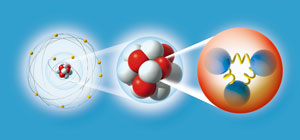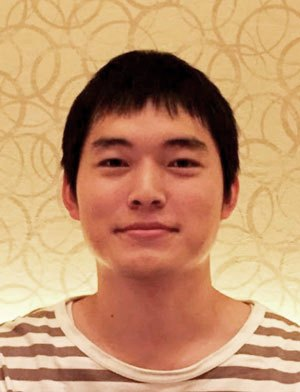1. Home
2. News & Publications
3. Research News

Feb. 22, 2019 Research Highlight Physics / Astronomy

# Machine learning algorithms could offer a glimpse into the heart of the proton

A computer algorithm that learns as it goes could hold the key to discovering what happens inside protons and neutronsFigure 1: The forces that hold an atom together (left) are well known, but what goes on inside the protons and neutrons (right) is harder to describe mathematically. © CLAUS LUNAU/SCIENCE PHOTO LIBRARY

By using cutting-edge computational techniques, RIKEN researchers have found a way to simplify the fiendishly difficult mathematics that describes the physics of fundamental particles1.

The electrical forces that bind the atomic nucleus and electrons to each other to form an atom are very well understood. Quantum electrodynamics (QED) combines relativity and quantum theory to describe how electrically charged particles interact in great detail. However, this theory does not apply to the individual subatomic particles themselves: a complete understanding of the physics that binds so-called quarks to make a proton or a neutron is still being developed.

Quantum chromodynamics, or QCD, attempts to describe the interaction between quarks using a concept known as ‘color’ charge, analogous to the electrical charge used as the basis for QED. But the mathematical tools used by particle physicists to simplify QED calculations are not useful in QCD, so alternatives need to be found.

One promising approach is the holographic model, which attempts to construct an equation of motion in a space that has an additional dimension. Just as a hologram recovers three-dimensional objects from a two-dimensional image, this model mimics the quantum behaviors of the original system.

“One of the most important features of the holographic model is that problems in a difficult quantum field theory like QCD can be mapped to equations of motion that are relatively easy to solve,” explains Akinori Tanaka from the RIKEN Center for Advanced Intelligence Project and the RIKEN Interdisciplinary Theoretical and Mathematical Sciences Program (iTHEMS).Akinori Tanaka and his colleagues have used machine learning to simplify calculations in quantum chromodynamics. © 2019 RIKEN

But building a holographic model to describe QCD is an enormous task, with so many variable parameters. “So far there is no mechanical method that can find a candidate of holographic model for a given quantum field theory,” says Tanaka. So he, along with Akio Tomiya from the RIKEN Brookhaven National Laboratory Research Center and their colleagues at Osaka University, tackled this problem using machine learning.

Machine learning is a computational technique that uses algorithms to build a mathematical model from a sample data set, which is then progressively improved.

“When we calculated the potential energy between quarks using our machine-learned holographic model, we found it qualitatively reproduces the same behavior seen in computer-simulated QCD calculations based on original quantum field theory,” says Tanaka.

This useful tool could help scientists solve knotty QCD problems, and hence lead to a better understanding of how nuclei behave during nuclear reactions, such as fission and fusion, and the formation of more exotic particles, such as those created in the Large Hadron Collider at CERN in Switzerland.

## Related contents

• 1. Hashimoto, K., Sugishita, S., Tanaka, A. & Tomiya, A. Deep learning and holographic QCD. Physical Review D 98, 106014 (2018). doi: 10.1103/PhysRevD.98.106014

Top